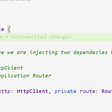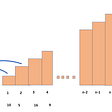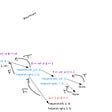When working with Binary Search Tree as a programmer you will be asked or required to traverse through the nodes. One concept you want to have in your arsenal is the “Breadth-First Search” technique. The basic method to this is to start from the root node and add all the additional nodes from the right or left to the queue. Once added to the queue it can be pushed to the final array. This technique is used for finding the shortest path in a graph.

How Does it Work

In order to start this method, we need to work off a Binary Tree. Create a variable and set it equal to the root node. Here’s what it looks like below.

`BFS(){            let currentNode = this.root}`

In addition, we will create two more variables and set both to empty arrays. The reason for this as explained earlier we will add the nodes to the queue, which will then be added to the result array. Furthermore, we will start off by pushing the root node to the queue. Here is a clear example below.

`BFS(){            let currentNode = this.root        let queue = []        let result = []                   queue.push(currentNode)}`

In this particular step, we initiate the traversing by looping through the queue. Create a while loop that takes an argument of queue.length. If the queue is empty the loop will not start.

`BFS(){            let currentNode = this.root        let queue = []        let result = []                   queue.push(currentNode)       while(queue.length){        }}`

Once the loop is initiated will set the variable we had earlier for the current node to queue.shift, which will remove the node in the queue.

`BFS(){            let currentNode = this.root        let queue = []        let result = []                   queue.push(currentNode)            while(queue.length){               currentNode = queue.shift()            }}`

We will add that current node value to the result. Here’s a better example below.

`BFS(){            let currentNode = this.root        let queue = []        let result = []                   queue.push(currentNode)         while(queue.length){               currentNode = queue.shift()               result.push(currentNode.value)        }}`

The last steps are to add the conditional for both left and right nodes. If the condition passes it will then be added to the queue. In addition, the last step is to return the final/result array.

`BFS(){            let currentNode = this.root        let queue = []        let result = []                   queue.push(currentNode)          while(queue.length){            currentNode = queue.shift()               result.push(currentNode.value)            if(currentNode.left) queue.push(currentNode.left)            if(currentNode.right) queue.push(currentNode.right)          }     return result}`

Thanks a lot for reading stay tuned for Depth First Search.

--

--

--

## More from Woodelin florveus

Love podcasts or audiobooks? Learn on the go with our new app.

## Data Structure: Bubble Sort## Top Ten JavaScript Interview Questions and Answers for beginners## CRUD application using NestJS and MongoDB## JavaScript Interview Questions (Part 2)## Why You Should Learn JavaScript First?## Angular: Understanding Life cycle hooks/events## Minimum cost to reach the top## 98. Validate Binary Search Tree## Everyday Things: Binary Search Trees & the Recursive Search Algorithm## How an Amazon Interview made me want to get out of my Introvert Comfort Zone